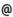## "Babes-Bolyai" University of Cluj-Napoca Faculty of Mathematics and Computer Science

 Special topics in modern mathematics (2)
 Code Semes-ter Hours: C+S+L Credits Type Section MO267 2 2+2+0 9 compulsory Algebră şi Geometrie - în limba engleză MO267 2 2+2+0 9 compulsory Analiză Reală şi Complexă - în limba engleză
 Teaching Staff in Charge
 Prof. DUCA Dorel, Ph.D., dducamath.ubbcluj.ro
 Aims Students will learn the methods and techniques of homological algebra.
 Content The topic of the ourse is Homological Algebra, which first arose as a language for describing topological propertiesof geometrical objects. Since then, it has expanded into subject on its own right, and its contemporary applications are many and diverse. A quick look at the Mathematics Subject Classification 2000 reveals application to Number Theory, Algebra and Differential Geometry, Lie Groups and Algebras, Finite Groups, Partial Differential Equations, Functional Analysis and Operator Theory. Therefore, the homological algebra methods must be in the toolbox of every mathematician. The aim of this cause is to introduce the basic concepts and techniques in the language of categories and functors, and to present examples coming from various fields. I. Simplicial sets. Triangulated spaces. Simplicial topological spaces. Sheaves II. Homology and Cohomology. Complexes and morphisms of complexes. Coefficient systems. The long exact sequence. Homotopy III. Examples. The Cech complex. The complex of singular chains. Homology and cohomology of groups. The de Rham complex. Homology and cohomology of Lie algebras. Hochschild (co)homology of algebras. Cyclic homology. The Koszul complex IV. Categories and functors. Categories. Functors and natural transformations. Equivalences of categories. Adjoint functors. Additive and abelian categories. V. Derived functors. Injective modules and projective modules. Resolutions.Derived functors Tor and Ext. Examples.
 References 1. S.I. GELFAND, Y.I. MANIN: Methods of Homological Algebra, Springer-Verlag 1998. 2. C. WEIBEL: An Introduction to Homological Algebra, Cambridge University Press, 1994. 3. H. CARTAN, S. EILENBERG: Homological Algebra, Princeton University Press, 1956. 4. P. HILTON, U. STAMMBACH: A Course in Homological Algebra, Springer-Verlag 1971. 5. S. MACLANE: Homology, Springer-Verlag 1963. 6. J. L. LODAY: Cyclic Homology, Springer-Verlag 1992.
 Assessment Homeworks. Essays. Exam.